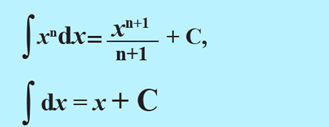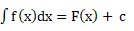AntiderivativesAntiderivatives

The reversed process of differentiation is known as integration. If f(x)

And F (x) are functions such thatF(x) = f(x) thenWhere c is known as the constant of integration.

Standard resultExamples

123